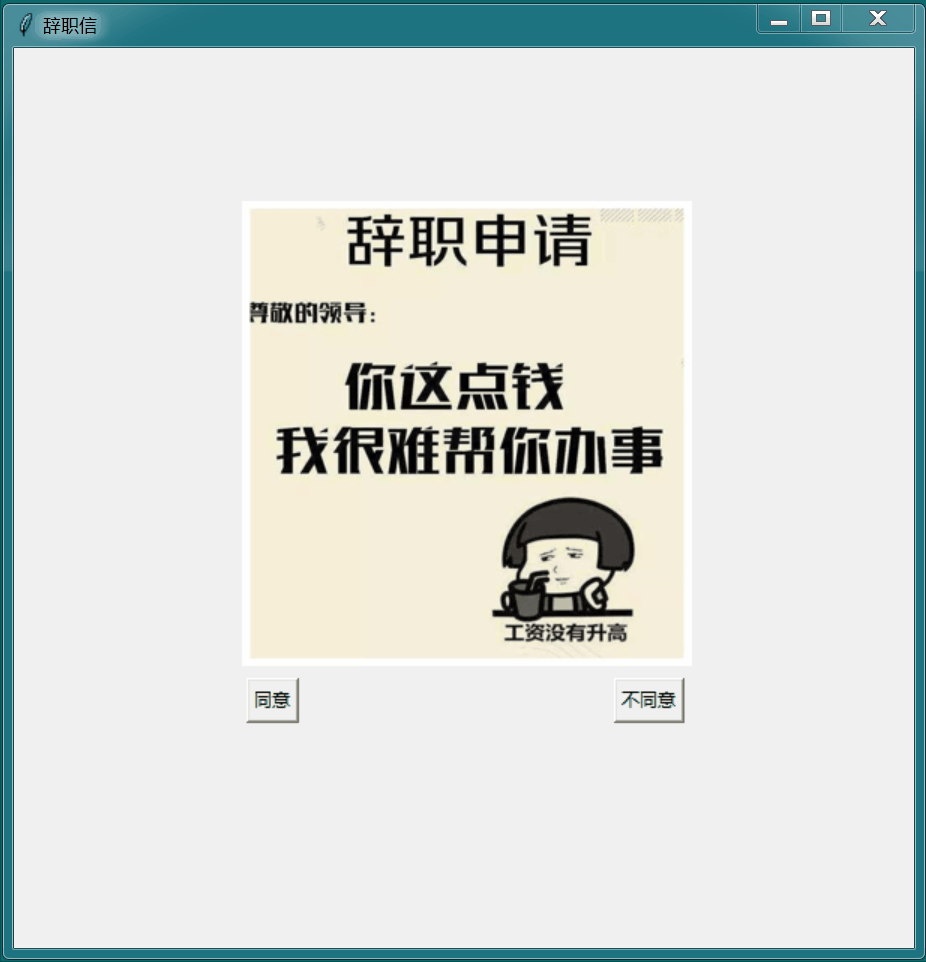# 我用 Python 把老板辞退了• 同意：点击同意弹出提示框，点击提示框上按钮可关闭整个窗口
• 不同意：点击不同意，不同意按钮移动，点击一次移动一次 ...
• 关闭窗口：点击 `X` 号关闭窗口，弹出羞辱提示框

``````def agree():
win = tk.Toplevel(window)
win.geometry("500x150+{}+{}".
format(int((screenwidth - width) / 2),
int((screenheight - height) / 2)))
win.title("辞职")
label = tk.Label(win, text="您耗子尾汁", font=("华文行楷", 20))
label.pack()
btn = tk.Button(win, text="滚出去", width=6, height=1, command=window.destroy)
btn.pack()

``````def disagree():
B2.place_forget()
B2.place(x=random.randint(100, 500), y=random.randint(100, 500))

``````def closeWindow():
messagebox.showinfo(title="不同意关不掉", message="关不掉吧，呵呵")